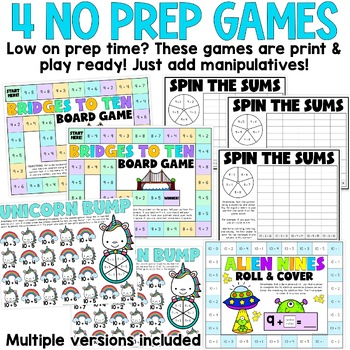Total:
\$0.00# Making 10 to Add 8 and 9 Games & Activities1st - 2nd, Homeschool
Subjects
Standards
Resource Type
Formats Included
• PDF
Pages
19 pages

#### Also included in

1. Use this bundle of addition fact fluency games & activities to enhance your basic addition to 20 skills. Strategies included: doubles & doubles plus one, ways to make 10, and using 10 to add 8 & 9.Looking for SUBTRACTION Fact Fluency Games & Activities? Find them HERE!Included in Dou
\$8.50
\$10.50
Save \$2.00

### Description

This is pack of printable games, activities, and centers are to help students learn how to make 10 to add. This resource is great to practice making ten to add for 1st grade or 2nd grade students. Use these activities in your math centers. This set of activities will help students learn to decompose problems with adding 8s and 9s to use 10 facts.

For example, students can take the problem 9 + 5 and "make a ten" and making the problem 10 + 4 ... I work with my students practicing this on a double ten frame.

Included in this resource:

• Helpful teacher teaching notes page
• Empty double ten frame for use with games
• Adding Nines Board Game - students race around a game board solving addition problems involving 9s
• Adding 8s & 9s Board Game - students race around a game board solving addition problems involving 9s
• Cookies & Milk Nines & Tens Memory Match - students match the 9 facts to the 10 facts (ex: 9 + 4 would match with 10 + 3)
• Spin Some Sums - students spin a spinner and solve the +8 or +9 fact (3 versions included)
• Using Tens Turtle Race - played similar to the deck of cards game "War"
• Using Tens Pizza Points - students choose a fact card and the solve it. The player with the larger sum gets a point for each round. The player with most points at the end of 10 rounds wins
• Adding Nines Roll & Cover - printable board game

Check out the Math Fact Fluency BUNDLE!

Find even more math fact fluency resources:

Total Pages
19 pages
N/A
Teaching Duration
N/A
Report this Resource to TpT
Reported resources will be reviewed by our team. Report this resource to let us know if this resource violates TpT’s content guidelines.

### Standards

to see state-specific standards (only available in the US).
Add and subtract within 20, demonstrating fluency for addition and subtraction within 10. Use strategies such as counting on; making ten (e.g., 8 + 6 = 8 + 2 + 4 = 10 + 4 = 14); decomposing a number leading to a ten (e.g., 13 - 4 = 13 - 3 - 1 = 10 - 1 = 9); using the relationship between addition and subtraction (e.g., knowing that 8 + 4 = 12, one knows 12 - 8 = 4); and creating equivalent but easier or known sums (e.g., adding 6 + 7 by creating the known equivalent 6 + 6 + 1 = 12 + 1 = 13).
Fluently add and subtract within 20 using mental strategies. By end of Grade 2, know from memory all sums of two one-digit numbers.